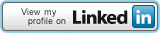## Connect Me## Pages

### HSEB based important C questions

Important questions to practice using C laguage based on HSEB approved text book.
WAP in C:
To ask length and breadth, and display the area and perimeter of a rectangle.
To ask a number and check whether it is Even or odd number.
To supply a number and check whether it is Prime or Composite number.
To supply a number and display whether it is a multiple of 7 or not.
To supply a number and print its factors. (12=1,2,3,4,6,12)
To supply a number and print the total number of factors of a user supplied number. (12=6)
Use for or while loop.
To ask the name and address of a person and print it for 25 times.
To enter the last number and print the sum of all numbers from 1 to that number.
To display the sum of first 10 multiples of 7.
To display the series: 1, 3, 5, 7, 9, 11, 13, 15, 17, 19.
To display multiplication table of 10 up to 100.
To display multiplication table of user entered number up to 10 times.
To display: 1, 2, 4, 7, 11, 16, 22, 29.
To display: 100, 85, 70, 55, 40, 25, 10.
To display: 1, 8, 27, 64, 125, ……. 1000.
To display: 2, 4, 8, 16, 32, 64, ……… 512.
To display: 2, 4, 6, 8, 10, 12,……………….. 50.
To display your favourite actor/actress name for 15 times.
To enter the marks of 5 subjects. Calculate total marks, percentage and division.
Use while or do-while loop.
To enter a number and find the sum of each digits. (123=6)
To enter a number and find the product of each digits.(234=24)
To enter a multi-digits number and display square of each digits. (123=1,4,9)
To enter a multi-digits number and display the sum of squares of each digits. (123=1+4+9=14)
To enter multi-digits number and display the revere of the supplied number. (1234=4321)

Ą